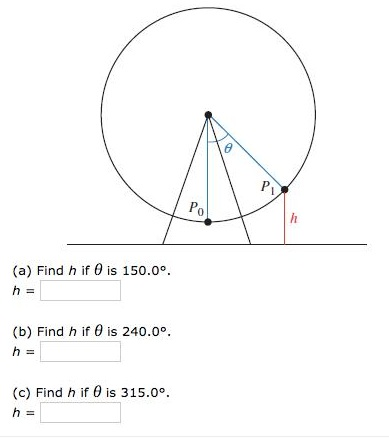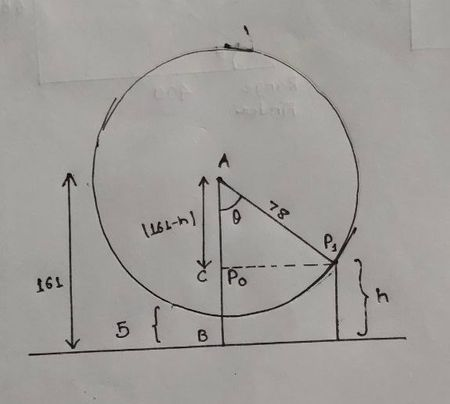# A Ferris wheel with a diameter of 156 feet was built in a city. The top of the wheel stands 161...

## Question:

A Ferris wheel with a diameter of 156 feet was built in a city. The top of the wheel stands 161 above the ground. Use the diagram in the figure below as a model of the wheel. Round your answers to the nearest number.## Ferris wheel:

A Ferris wheel is an amusement ride consisting of a large vertical revolving wheel with passenger seats attached to the rim of the giant wheel and the Ferris wheel is attached to the ground rigidly through its center.

Here, we will be using trigonometric identities to solve for the value of h at different angles given.

Given:

Diameter of Ferris wheel = 156 feet

The radius of the Ferris wheel is 78 feet

The distance between the {eq}p_{o}\; {/eq} and ground level = 161 - 156 = 5 feet

Distance between the center and the ground level = 161 feetFrom, the figure:

AC = AB - h

AC = 161 - h

(a)

{eq}\theta = 150^{\circ}\\ \text{Using trigonometric formula}\\ \cos \theta = \frac{161 - h}{78}\\ \cos 150^{\circ} = \frac{161 - h}{78} - 67.55 = 161 - h\\ h = 228.55\; feet {/eq}

(b)

{eq}\theta = 240^{\circ}\\ \text{Using trigonometric formula}\\ \cos \theta = \frac{161 - h}{78}\\ \cos 240^{\circ} = \frac{161 - h}{78}\\ -39 = 161 - h\\ h = 200\; feet {/eq}

(c)

{eq}\theta = 315^{\circ}\\ \text{Using trigonometric formula}\\ \cos \theta = \frac{161 - h}{78}\\ \cos 315^{\circ} = \frac{161 - h}{78}\\ 55.154 = 161 - h\\ h = 105.84\; feet {/eq}